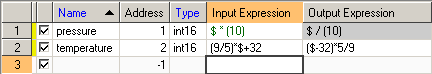### 5.3.6. Tag Expression

Tag Expression is simple Javascript synyax that defines a formula to alter the value of the tag.

For ordinary scaling requirement, user only need to enter any numerical number as needed. For instance, divided by a thousand can be entered as 0.001, or scaling factor like multiplied by a thousand can be entered as 1000.

User will soon realize the entered number will be automatically added with some syntax with \$ symbol when the cursor is away from the field. As per the examples above, entering 0.001 will change to \$*(0.001), and entering 1000 will change to \$*(1000).Note

When the cursor is back to the expression column, the originally entered number will return and ready for update.Expression for scaling/calculationTip

The source value can be omitted by adding \$*0+ in front of the code. And the auto scaling feature can be removed by entering \$+0.

Example 5.13. Expression without tag linking

User may do any complicated engineering unit conversion like °C to °F. To do this, enter the following input expression:

`(9/5) * \$ +32`

And enter the following output expression:

`(\$-32) *5 /9`

Another practical PLC raw value linear scaling example, if raw value of 819 represent 10°C, and 4095 represent 60°C, then the input expression shall be entered as:

`((60-10) * (\$-819) / (4095-819)) +10`

Conversely the output expression can be entered as:

`((\$ -10) * (4095-819) / (60-10)) +819`

Example 5.14. Expression with tag linking

User can even link several tag inputs into conversion. For a flow calculation that takes frequency as input, enter the following as input expression:

`(\$ / \$KFac) *3600 * \$MFac`

Whereby MFac and KFac are tags for Meter Factor and K-Factor input respectively.

Example 5.15. Expression with JavaScript function

User may also add fool-proof interlock by clipping the output within High and Low limits by entering the following output expression, whereby LIM_LO and LIM_HI clipped the desired output range.

`Math.max(LIM_LO, Math.min(LIM_HI, \$))`

For instance, entering the following expression will prevent operator from sending anything less than zero or bigger than hundred to the external device.

`Math.max(0,Math.min(100,\$))`

For the sake of explanation, this Expression has the similar effect of entering the following ternary expression.

`(\$ > LIM_HI ? LIM_HI : \$) < LIM_LO ? LIM_LO : \$`

Example 5.16. Performing bitwise operation with expression

It's also very common to perform bitwise operation with external devices in which the Tag Expression can do the magic.

To read LSB and MSB in one 16 bit integer, the expression can be entered as \$&1 and \$&65536 respectively (or reversely).

To send bits out to an integer, can be written as:

`\$0 + \$b0*1 + \$b1*2 + \$b2*4 + \$b3*8 + \$b4*16 + \$b5*32 + \$b6*64 + \$b7*128 + \$b8*256 + \$b9*512 + \$bA*1024 + \$bB*2048 + \$bC*4096 + \$bD*8192 + \$bE*16384 + \$bF*32768`

whereby b0...b9 and bA...bF are the 16 boolean tags representing individual bit.

System would expect some formular to be entered in . Simply enter single \$ symbol if no further manipulation of the value needed.Important

JavaScript doesn't perform bitwise operation beyond 32 bit. Using int64 or uint64 in bitwise operation will not produce expected output.